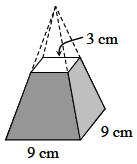Home > CCG > Chapter 11 > Lesson 11.2.2 > Problem11-100

11-100.The solid at right is an example of a truncated pyramid. It is formed by slicing and removing the top of a pyramid so that the slice is parallel to the base of the pyramid. If the original height of the square-based pyramid at right was $12$ cm, find the volume of this truncated pyramid. (Hint: You may find your results from problem 11‑86 useful.)

Find the volume of the large pyramid.

$V = \frac{1}{3} (\text{volume of corresponding prism})$

$V = \frac{1}{3}(9 \cdot 9 \cdot 12) = 324\text{ cm}^3$

The side of the small pyramid is $\frac{1}{3}$ the side of the large pyramid, because $3$ is $\frac{1}{3}$ of $9$

So, the lsf is $\frac{1}{3}$.

Find the volume of the small pyramid.

$\text{(volume larger)(lsf)}^3 = \text{volume smaller}$

$324 \cdot \left(\frac{1}{3}\right)^3 = 324 \cdot \frac{1}{27} = 12\text{ cm}^3$

Subtract the volume of the smaller pyramid from the volume of the larger pyramid.

$324 − 12$

$312\text{ cm}^3$